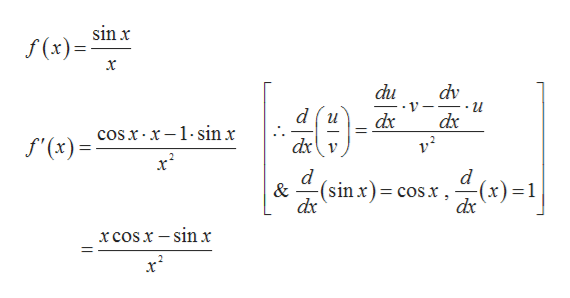# f(x)=sinx/x  ,  c= π/3what does f'(c)=

Question

f(x)=sinx/x  ,  c= π/3

what does f'(c)=

check_circleExpert Solution
Step 1

Given,

Step 2

Differentiating the given function b...help_outlineImage Transcriptionclose(x)sinx x du dv и du dx dx cos x x-1-sin x dxv f'(x) d (sinx)= cosx, (x)1 & dx xcosx - sin x fullscreen

### Want to see the full answer?

See Solution

#### Want to see this answer and more?

Solutions are written by subject experts who are available 24/7. Questions are typically answered within 1 hour*

See Solution
*Response times may vary by subject and question
Tagged in

### Derivative